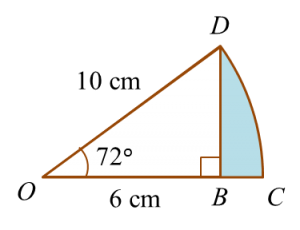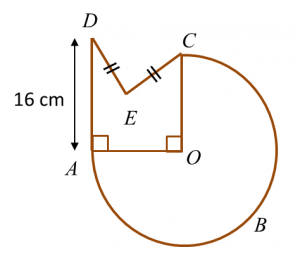# 10.2.2 Circles I, PT3 Focus Practice

                       
Question 6:
       In the diagram below, CD is an arc of a circle with centre O.Determine the area of the shaded region.
                                                    
 
 Solution:

                                             
      
    
      
                   
 Question 7:
 In diagram below, ABC is a semicircle with centre O.Calculate the area, in cm2 , of the shaded region.
                                     
 
   Solution:
                                         
 
 
                   
 Question 8:
 In diagram below, ABC is an arc of a circle centre OThe radius of the circle is 14 cm and AD = 2 DE.
   Calculate the perimeter, in cm, of the whole diagram.
                                       
 
    Solution:
                                       
 
 
 [adinserter block="3"]                
Question 9:
 
In diagram below, KLMN is a square and KLON is a quadrant of a circle with centre K.Calculate the area, in cm2, of the coloured region.
                     
 
 Solution:
               
 
 
 
 [adinserter block="3"]                
Question 10:
 
Diagram below shows two quadrants, AOC and EOD with centre O.Sector AOB and sector BOC have the same area.
 Calculate the area, in cm2, of the coloured region.
                     
 
 Solution: